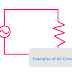Here, is the common examples of AC Circuits are,
1. Household Wirings
2. Railway Electrical System
3. Electrical Power Transmission and Distribution
4. Star Delta Starter Circuit
5. DOL Starter Circuit
6. Series Reactor Circuit
7. Alternator Parallel Operation Circuit
8. Transformer Parallel Operation Circuit
9. Electrical Crane or Elevator Circuit
10. Impedance Control Circuit
11. Power Factor Controller Circuit

AC circuits are those where alternating current flows from the power source to load and through all the components. Alternating current or AC has changing magnitude and polarity characteristics. So, there is a lot of difference between an AC circuit and a DC circuit. In the AC circuit, there is no fixed polarity such as positive or negative. The most common AC circuits are used in single-phase, double-phase, and three-phase formats. In a single-phase AC circuit there are two wires available phase and neutral whereas in a three-phase AC circuit R, Y, B and in a four-wire AC circuit R, Y, B, N.

Now, let's talk about the common examples of AC circuits listed in the above section.

The first one is the Household wiring. In our house, and office all the lights, fans, and other loads are operated with an AC supply provided by a utility source. So our household wiring is an example of an AC Circuit.

The railway electrification system is powered by AC Supply. All the power transmission and distribution circuits for the railway system are AC circuits.

We know that the electrical power transmission and distribution system also works with AC supply(single-phase and three-phase).

The AC motor starter circuits such as the star delta circuit, and the DOL starter circuit also work with AC supply. Star delta starter is used for high power motors whereas the DOL starter is used for low power motors.

The series reactor is built with inductors. The main function of this series reactor circuit is to block the unwanted signal and power. This is achieved by the reactance property of the inductor. And we know that the inductor will show its inductive reactance property with AC supply only. If it is supplied by a DC supply there will be no reactance effect occur.

Multiple alternators are connected in parallel to increase the power generation capacity of the system and backup system. These parallel operation circuits also contain some important components. As the alternator generates an alternating power supply, it can be called an example of an AC Circuit.

Same the multiple transformers are connected in parallel for some applications. The transformer is an AC machine and it works with AC supply only.

The last one is the power factor controller circuit. We know that the concept of power factor comes in AC circuits only. The power factor controller circuit is built with capacitors, thyristors, and so many other components. They all are worked with alternating current. But if the power factor controller is a digital circuit then its control circuit is supplied by a DC source using a rectifier circuit. But in the main operation of the power factor control, DC supply does not involve.

10+ Examples of AC CircuitsReviewed by Author on May 19, 2022 Rating: 5